• 梯度下降法python实现
千次阅读
2022-02-28 21:52:31

# 前言

梯度下降算法 Gradient Descent GD是沿梯度下降的方向连续迭代逼近求最小值的过程，本文将实现以下梯度下降算法的python实现。

• 简单梯度下降算法
• 批量梯度下降算法
• 随机梯度下降算法

# 简单梯度下降算法

简单梯度下降算法的核心就是先求出目标函数的导数 g k g_k ,然后利用简单随机梯度西江算法公式迭代求最小值。
x k + 1 = x k − g k ∗ r k x_{k+1}=x_k-g_k*r_k 

• x k + 1 x_{k+1} 下一步位置
• x k x_{k} 当前位置
• g k g_k 为梯度
• r k r_k 学习率，步长

有一个目标函数， f ( x ) = x 2 f(x)=x^2 ,一元函数的导数就是该曲线某一点切线的斜率，导数越大，该点的斜率越大，下降越快
F ′ = 2 x F^{'}=2x 这就是梯度， r k r_k 控制着梯度下降前进距离，太大太小都不行。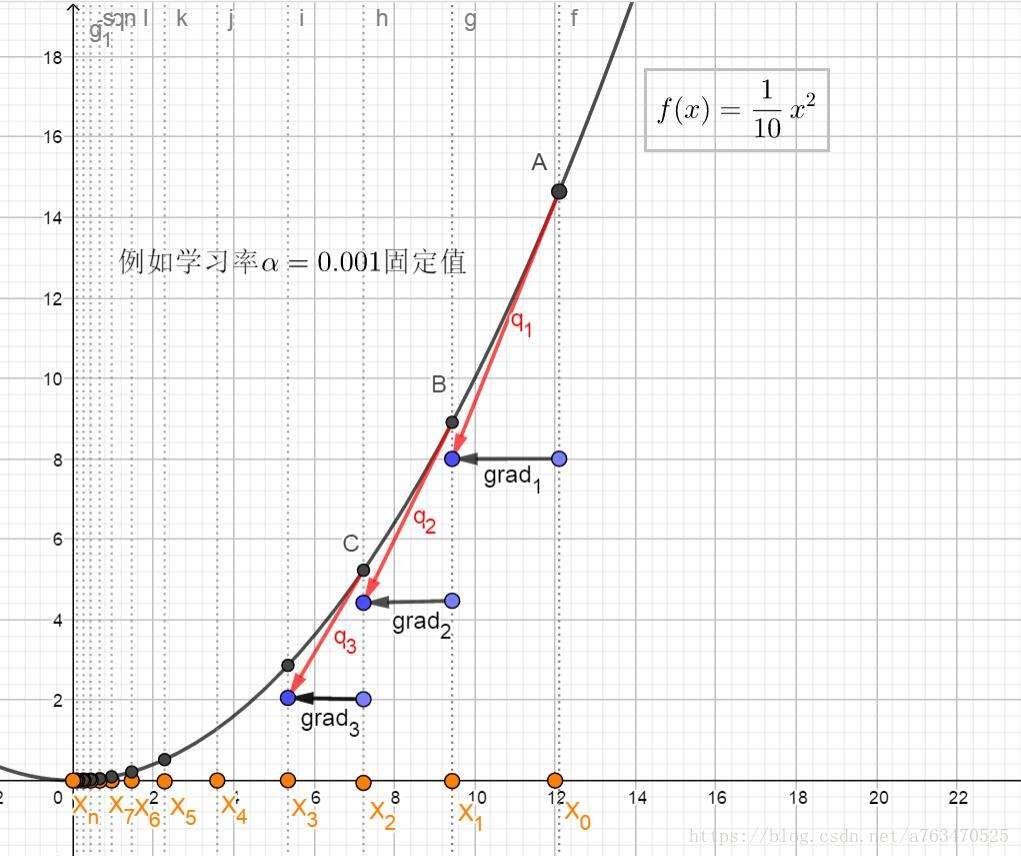## 代码

import numpy as np
import matplotlib.pyplot as plt

# 定义目标函数 f(x)=x**2+1
def f(x):
return np.array(x)**2 + 1

# 对目标函数求导 d(x)=x*2
def d1(x):
return x * 2

def Gradient_Descent_d1(current_x = 0.1,learn_rate = 0.01,e = 0.001,count = 50000):
# current_x initial x value
# learn_rate 学习率
# e error
# count number of iterations
for i in range(count):
grad = d1(current_x) # 求当前梯度
if abs(grad) < e: # 梯度收敛到控制误差内
break # 跳出循环
current_x = current_x - grad * learn_rate # 一维梯度的迭代公式
print("第{}次迭代逼近值为{}".format(i+1,current_x))

print("最小值为：",current_x)
print("最小值保存小数点后6位：%.6f"%(current_x))
return current_x

# 显示目标函数曲线及梯度下降最小值毕竟情况
def ShowLine(min_X,max_Y):
x = [x for x in range(10)] + [x * (-1) for x in range(1,10)]
x.sort()
print(x)
plt.plot(x,f(x))
plt.plot(min_X,max_Y,'ro')
plt.show()

minValue = Gradient_Descent_d1(current_x = 0.1,learn_rate = 0.01,e = 0.001,count = 50000)
minY = f(minValue)
print('目标函数最小值约为：',minY)
ShowLine(minValue,minY)


## 输出

第1次迭代逼近值为0.098
第2次迭代逼近值为0.09604
第3次迭代逼近值为0.0941192
第4次迭代逼近值为0.092236816
第5次迭代逼近值为0.09039207968
第6次迭代逼近值为0.08858423808640001
第7次迭代逼近值为0.08681255332467201
第8次迭代逼近值为0.08507630225817857
第9次迭代逼近值为0.08337477621301499
第10次迭代逼近值为0.08170728068875469
第11次迭代逼近值为0.0800731350749796
第12次迭代逼近值为0.07847167237348
第13次迭代逼近值为0.0769022389260104
第14次迭代逼近值为0.0753641941474902
第15次迭代逼近值为0.07385691026454039
第16次迭代逼近值为0.07237977205924959
第17次迭代逼近值为0.0709321766180646
第18次迭代逼近值为0.06951353308570331
第19次迭代逼近值为0.06812326242398924
第20次迭代逼近值为0.06676079717550945
第21次迭代逼近值为0.06542558123199926
第22次迭代逼近值为0.06411706960735927
第23次迭代逼近值为0.06283472821521209
第24次迭代逼近值为0.061578033650907846
第25次迭代逼近值为0.06034647297788969
第26次迭代逼近值为0.059139543518331894
第27次迭代逼近值为0.05795675264796526
第28次迭代逼近值为0.056797617595005956
第29次迭代逼近值为0.05566166524310584
第30次迭代逼近值为0.05454843193824372
第31次迭代逼近值为0.05345746329947885
第32次迭代逼近值为0.05238831403348927
第33次迭代逼近值为0.05134054775281949
第34次迭代逼近值为0.050313736797763096
第35次迭代逼近值为0.04930746206180783
第36次迭代逼近值为0.04832131282057168
第37次迭代逼近值为0.047354886564160245
第38次迭代逼近值为0.046407788832877044
第39次迭代逼近值为0.0454796330562195
第40次迭代逼近值为0.04457004039509511
第41次迭代逼近值为0.0436786395871932
第42次迭代逼近值为0.04280506679544934
第43次迭代逼近值为0.041948965459540355
第44次迭代逼近值为0.041109986150349546
第45次迭代逼近值为0.040287786427342556
第46次迭代逼近值为0.0394820306987957
第47次迭代逼近值为0.03869239008481979
第48次迭代逼近值为0.03791854228312339
第49次迭代逼近值为0.03716017143746093
第50次迭代逼近值为0.036416968008711706
第51次迭代逼近值为0.035688628648537474
第52次迭代逼近值为0.034974856075566725
第53次迭代逼近值为0.03427535895405539
第54次迭代逼近值为0.03358985177497428
第55次迭代逼近值为0.032918054739474796
第56次迭代逼近值为0.0322596936446853
第57次迭代逼近值为0.03161449977179159
第58次迭代逼近值为0.03098220977635576
第59次迭代逼近值为0.030362565580828647
第60次迭代逼近值为0.029755314269212074
第61次迭代逼近值为0.029160207983827832
第62次迭代逼近值为0.028577003824151275
第63次迭代逼近值为0.028005463747668248
第64次迭代逼近值为0.027445354472714883
第65次迭代逼近值为0.026896447383260587
第66次迭代逼近值为0.026358518435595377
第67次迭代逼近值为0.025831348066883468
第68次迭代逼近值为0.0253147211055458
第69次迭代逼近值为0.024808426683434883
第70次迭代逼近值为0.024312258149766185
第71次迭代逼近值为0.02382601298677086
第72次迭代逼近值为0.023349492727035442
第73次迭代逼近值为0.02288250287249473
第74次迭代逼近值为0.022424852815044836
第75次迭代逼近值为0.02197635575874394
第76次迭代逼近值为0.02153682864356906
第77次迭代逼近值为0.02110609207069768
第78次迭代逼近值为0.020683970229283727
第79次迭代逼近值为0.020270290824698053
第80次迭代逼近值为0.019864885008204092
第81次迭代逼近值为0.01946758730804001
第82次迭代逼近值为0.019078235561879212
第83次迭代逼近值为0.01869667085064163
第84次迭代逼近值为0.018322737433628795
第85次迭代逼近值为0.017956282684956217
第86次迭代逼近值为0.017597157031257093
第87次迭代逼近值为0.017245213890631952
第88次迭代逼近值为0.016900309612819315
第89次迭代逼近值为0.01656230342056293
第90次迭代逼近值为0.01623105735215167
第91次迭代逼近值为0.015906436205108634
第92次迭代逼近值为0.015588307481006461
第93次迭代逼近值为0.015276541331386333
第94次迭代逼近值为0.014971010504758606
第95次迭代逼近值为0.014671590294663434
第96次迭代逼近值为0.014378158488770165
第97次迭代逼近值为0.014090595318994762
第98次迭代逼近值为0.013808783412614867
第99次迭代逼近值为0.01353260774436257
第100次迭代逼近值为0.013261955589475318
第101次迭代逼近值为0.012996716477685811
第102次迭代逼近值为0.012736782148132095
第103次迭代逼近值为0.012482046505169453
第104次迭代逼近值为0.012232405575066064
第105次迭代逼近值为0.011987757463564744
第106次迭代逼近值为0.01174800231429345
第107次迭代逼近值为0.01151304226800758
第108次迭代逼近值为0.01128278142264743
第109次迭代逼近值为0.01105712579419448
第110次迭代逼近值为0.01083598327831059
第111次迭代逼近值为0.010619263612744378
第112次迭代逼近值为0.01040687834048949
第113次迭代逼近值为0.010198740773679701
第114次迭代逼近值为0.009994765958206107
第115次迭代逼近值为0.009794870639041985
第116次迭代逼近值为0.009598973226261145
第117次迭代逼近值为0.009406993761735923
第118次迭代逼近值为0.009218853886501205
第119次迭代逼近值为0.009034476808771182
第120次迭代逼近值为0.008853787272595759
第121次迭代逼近值为0.008676711527143843
第122次迭代逼近值为0.008503177296600965
第123次迭代逼近值为0.008333113750668945
第124次迭代逼近值为0.008166451475655567
第125次迭代逼近值为0.008003122446142456
第126次迭代逼近值为0.007843059997219607
第127次迭代逼近值为0.007686198797275215
第128次迭代逼近值为0.00753247482132971
第129次迭代逼近值为0.0073818253249031155
第130次迭代逼近值为0.007234188818405053
第131次迭代逼近值为0.0070895050420369515
第132次迭代逼近值为0.006947714941196213
第133次迭代逼近值为0.006808760642372289
第134次迭代逼近值为0.006672585429524843
第135次迭代逼近值为0.006539133720934346
第136次迭代逼近值为0.006408351046515659
第137次迭代逼近值为0.006280184025585346
第138次迭代逼近值为0.006154580345073639
第139次迭代逼近值为0.0060314887381721655
第140次迭代逼近值为0.005910858963408722
第141次迭代逼近值为0.005792641784140548
第142次迭代逼近值为0.005676788948457737
第143次迭代逼近值为0.005563253169488583
第144次迭代逼近值为0.005451988106098811
第145次迭代逼近值为0.005342948343976835
第146次迭代逼近值为0.005236089377097298
第147次迭代逼近值为0.005131367589555352
第148次迭代逼近值为0.005028740237764245
第149次迭代逼近值为0.00492816543300896
第150次迭代逼近值为0.004829602124348781
第151次迭代逼近值为0.004733010081861806
第152次迭代逼近值为0.004638349880224569
第153次迭代逼近值为0.004545582882620078
第154次迭代逼近值为0.004454671224967677
第155次迭代逼近值为0.004365577800468323
第156次迭代逼近值为0.004278266244458957
第157次迭代逼近值为0.004192700919569778
第158次迭代逼近值为0.004108846901178382
第159次迭代逼近值为0.004026669963154815
第160次迭代逼近值为0.003946136563891718
第161次迭代逼近值为0.003867213832613884
第162次迭代逼近值为0.0037898695559616066
第163次迭代逼近值为0.0037140721648423742
第164次迭代逼近值为0.003639790721545527
第165次迭代逼近值为0.0035669949071146165
第166次迭代逼近值为0.003495655008972324
第167次迭代逼近值为0.0034257419087928777
第168次迭代逼近值为0.00335722707061702
第169次迭代逼近值为0.00329008252920468
第170次迭代逼近值为0.0032242808786205864
第171次迭代逼近值为0.003159795261048175
第172次迭代逼近值为0.0030965993558272112
第173次迭代逼近值为0.003034667368710667
第174次迭代逼近值为0.0029739740213364538
第175次迭代逼近值为0.0029144945409097247
第176次迭代逼近值为0.0028562046500915303
第177次迭代逼近值为0.0027990805570896997
第178次迭代逼近值为0.0027430989459479057
第179次迭代逼近值为0.0026882369670289475
第180次迭代逼近值为0.0026344722276883687
第181次迭代逼近值为0.0025817827831346014
第182次迭代逼近值为0.0025301471274719093
第183次迭代逼近值为0.002479544184922471
第184次迭代逼近值为0.0024299533012240217
第185次迭代逼近值为0.0023813542351995413
第186次迭代逼近值为0.0023337271504955503
第187次迭代逼近值为0.0022870526074856394
第188次迭代逼近值为0.0022413115553359267
第189次迭代逼近值为0.0021964853242292083
第190次迭代逼近值为0.002152555617744624
第191次迭代逼近值为0.0021095045053897317
第192次迭代逼近值为0.002067314415281937
第193次迭代逼近值为0.0020259681269762984
第194次迭代逼近值为0.0019854487644367725
第195次迭代逼近值为0.001945739789148037
第196次迭代逼近值为0.0019068249933650763
第197次迭代逼近值为0.0018686884934977748
第198次迭代逼近值为0.0018313147236278192
第199次迭代逼近值为0.0017946884291552628
第200次迭代逼近值为0.0017587946605721575
第201次迭代逼近值为0.0017236187673607144
第202次迭代逼近值为0.0016891463920135
第203次迭代逼近值为0.00165536346417323
第204次迭代逼近值为0.0016222561948897654
第205次迭代逼近值为0.0015898110709919701
第206次迭代逼近值为0.0015580148495721307
第207次迭代逼近值为0.001526854552580688
第208次迭代逼近值为0.0014963174615290743
第209次迭代逼近值为0.0014663911122984928
第210次迭代逼近值为0.001437063290052523
第211次迭代逼近值为0.0014083220242514724
第212次迭代逼近值为0.001380155583766443
第213次迭代逼近值为0.0013525524720911142
第214次迭代逼近值为0.0013255014226492918
第215次迭代逼近值为0.001298991394196306
第216次迭代逼近值为0.00127301156631238
第217次迭代逼近值为0.0012475513349861323
第218次迭代逼近值为0.0012226003082864098
第219次迭代逼近值为0.0011981483021206816
第220次迭代逼近值为0.001174185336078268
第221次迭代逼近值为0.0011507016293567027
第222次迭代逼近值为0.0011276875967695687
第223次迭代逼近值为0.0011051338448341773
第224次迭代逼近值为0.0010830311679374937
第225次迭代逼近值为0.001061370544578744
第226次迭代逼近值为0.001040143133687169
第227次迭代逼近值为0.0010193402710134258
第228次迭代逼近值为0.0009989534655931573
第229次迭代逼近值为0.000978974396281294
第230次迭代逼近值为0.0009593949083556683
第231次迭代逼近值为0.000940207010188555
第232次迭代逼近值为0.0009214028699847839
第233次迭代逼近值为0.0009029748125850882
第234次迭代逼近值为0.0008849153163333864
第235次迭代逼近值为0.0008672170100067186
第236次迭代逼近值为0.0008498726698065842
第237次迭代逼近值为0.0008328752164104526
第238次迭代逼近值为0.0008162177120822435
第239次迭代逼近值为0.0007998933578405986
第240次迭代逼近值为0.0007838954906837866
第241次迭代逼近值为0.0007682175808701109
第242次迭代逼近值为0.0007528532292527087
第243次迭代逼近值为0.0007377961646676545
第244次迭代逼近值为0.0007230402413743014
第245次迭代逼近值为0.0007085794365468154
第246次迭代逼近值为0.0006944078478158791
第247次迭代逼近值为0.0006805196908595615
第248次迭代逼近值为0.0006669092970423702
第249次迭代逼近值为0.0006535711111015229
第250次迭代逼近值为0.0006404996888794924
第251次迭代逼近值为0.0006276896951019025
第252次迭代逼近值为0.0006151359011998645
第253次迭代逼近值为0.0006028331831758672
第254次迭代逼近值为0.0005907765195123498
第255次迭代逼近值为0.0005789609891221028
第256次迭代逼近值为0.0005673817693396607
第257次迭代逼近值为0.0005560341339528675
第258次迭代逼近值为0.0005449134512738102
第259次迭代逼近值为0.0005340151822483339
第260次迭代逼近值为0.0005233348786033672
第261次迭代逼近值为0.0005128681810312999
第262次迭代逼近值为0.0005026108174106739
第263次迭代逼近值为0.0004925586010624604
最小值为： 0.0004925586010624604
最小值保存小数点后6位：0.000493
目标函数最小值约为： 1.0000002426139756
[-9, -8, -7, -6, -5, -4, -3, -2, -1, 0, 1, 2, 3, 4, 5, 6, 7, 8, 9]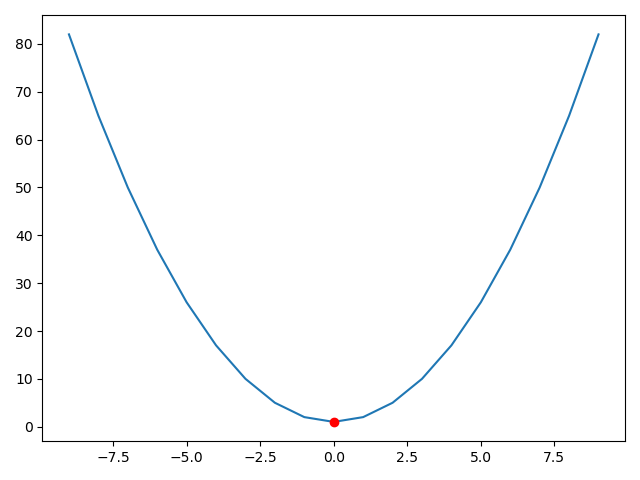# 批量梯度下降算法

批量梯度下降，Batch Gradient Descent BGD 算法是对所有的样本数据进行梯度迭代计算，这里“所有”考虑了非凹凸函数（存在多个局部极大值或极小值的情况）
损失函数
J ( θ ) = 1 2 n Σ i = 1 n ( h θ i − y i ) 2 J(\theta)=\frac{1}{2n}\Sigma_{i=1}^{n}(h_{\theta}^{i}-y_i)^2

• n n 是样本个数
• 1/2求偏导时可以相互抵消
• x i , y i x^i,y^i 是第i个样本的 ( x , y ) (x,y) 坐标值
假设函数的公式为：
h θ ( x i ) = θ 0 + θ 1 x 1 i + θ 2 x 2 i + ⋅ ⋅ ⋅ + θ n x n i h_{\theta}(x^i)=\theta_0+\theta_1x_1^{i}+\theta_2x_2^{i}+···+\theta_nx_n^{i}
批量梯度是值在对全样本数据（任意维度）计算梯度时，通过计算损失函数求偏导得到梯度计算公式
$∇ θ J ( θ ) = ∂ J ( θ ) ∂ θ j = 1 n Σ i = 1 n ( h θ i − y i ) 2 x j i \nabla_\theta J(\theta)=\frac{\partial J(\theta)}{\partial \theta_j}=\frac{1}{n} \Sigma_{i=1}^{n}(h_{\theta}^{i}-y_i)^2x_j^{i} i = 1 , 2 , ⋅ ⋅ ⋅ n i=1,2,···n 表示样本数， j = 0 , 1 表 示 特 征 数 j=0,1表示特征数 批量迭代公式： θ = θ − μ ⋅ ∇ θ J ( θ ) \theta=\theta-\mu· \nabla_\theta J(\theta) # 随机梯度下降算法 随机梯度下降（Stochastic Gradient Descent SGD）通过每次梯度迭代随机采用一个样本数量，最后逼近极值得出近似预测结果。损失函数计算公式： J ( θ ) = 1 2 ( h θ ( x i ) − y i ) 2 J(\theta)=\frac{1}{2}(h_\theta(x^i)-y^i)^2 特别指出：随机梯度下降算法每次迭代只对一个样本数据进行计算，与批量梯度下降算法相比，损失函数迭代一次无须求所有样本的值，因此不需要求均值。$ ∇ θ J ( θ ) = ∂ J ( θ ) ∂ θ j = ( h θ i − y i ) 2 x j i \nabla_\theta J(\theta)=\frac{\partial J(\theta)}{\partial \theta_j}=(h_{\theta}^{i}-y_i)^2x_j^{i}
迭代公式：
θ = θ − μ ⋅ ∇ θ J ( θ ) \theta=\theta-\mu·\nabla_\theta J(\theta)python 机器学习
更多相关内容
• 随机梯度下降法的做法是使用一下表达式（我前面的博客有讲到公式推理过程）来更新参数，表达式中的k 是随机选择的 现在有了训练数据的矩阵X，把行的顺序随机地予以调整，然后重复应用更新表达式就行了。 import...

随机梯度下降法的做法是使用一下表达式（我前面的博客有讲到公式推理过程）来更新参数，表达式中的k 是随机选择的

现在有了训练数据的矩阵X，把行的顺序随机地予以调整，然后重复应用更新表达式就行了。

import numpy as np
import matplotlib.pyplot as plt
#读入训练数据
train = np.loadtxt('D:/NoteBook/sourcecode-cn/click.csv', delimiter=',', skiprows=1)
train_x = train[:,0]
train_y = train[:,1]
#标准化
mu = train_x.mean()
sigma = train_x.std()
def standardize(x):
return (x - mu)/ sigma
train_z = standardize(train_x)
#用随机数对参数初始化
theta = np.random.rand(3)
#创建训练数据矩阵
def to_matrix(x):
return np.vstack([np.ones(x.shape), x, x**2]).T
X = to_matrix(train_z)
#预测函数
def f(x):
return np.dot(x, theta)
#均方误差的历史纪录
errors = []
#误差的差值
diff = 1
#学习率
ETA = 1e-3
#均方误差
def MSE(x, y):
return (1 / x.shape) * np.sum((y- f(x)) ** 2)
#重复学习
errors.append(MSE(X, train_y))
while diff > 1e-2:
#为了调整训练的顺序，准备随机的序列
p = np.random.permutation(X.shape)#shape是行数，np.random.permutation是序列进行随机排序，对于一个多维的输入，只是在第一维上进行了随机排序。对矩阵来说，是对行数据进行随机排序。
#随机取出训练数据，使用随机梯度下降法更新参数
for x, y in zip(X[p,:], train_y[p]):  #X[p,:]第一维单取下标p，第二维取全部，就是下标p的全部数据作为训练数据，对应第p个数据的y值为实际值。
theta = theta - ETA * (f(x) - y)*x
#计算与上一次误差的差值
errors.append(MSE(X, train_y))
diff = errors[-2] - errors[-1]
x = np.linspace(-3, 3, 100)
plt.plot(train_z, train_y, 'o')
plt.plot(x, f(to_matrix(x)))
plt.show()

打印的结果如下图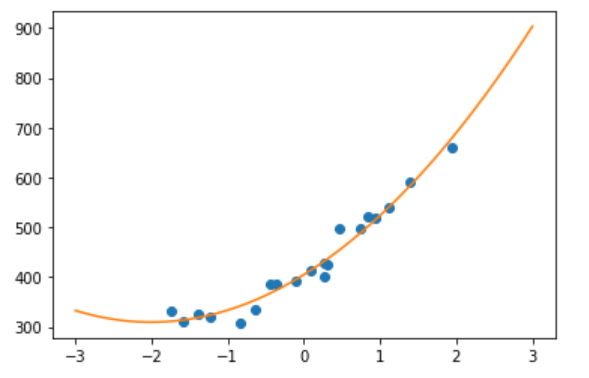多重回归的实现，也可以像多项式回归时那样使用矩阵，不过要注意对多重回归的变量进行标准化时，必须对每个参数都进行标准化。如果有变量，就要分别使用每个变量的平均值和标准差进行标准化。

展开全文python 机器学习
• 主要为大家详细介绍了python实现随机梯度下降法，具有一定的参考价值，感兴趣的小伙伴们可以参考一下
• 本文主要以线性回归算法损失函数求极小值来说明如何使用梯度下降算法并给出python实现。若有不正确的地方，希望读者能指出。  梯度下降 梯度下降原理：将函数比作一座山，我们站在某个山坡上，往四周看，从哪个方向...
• 在机器学习中,我们在求最小值时使用梯度下降法,求最大值时使用梯度上升法. 为了尽快的得到最小值或者最大值,我们尽量让每一步运算的变化率都足够大,因此,在每一次运算时,我们要使函数变化的方向与梯度相同或相反,即...
• m = 100000 x = np.random.normal(size = m) X = x.reshape(-1, 1) y = 4.0 * x + 3.0 + np.random.normal(0 ,3, size = m) 。。。随机梯度下降
• 点击上方“小白学视觉”，选择加"星标"或“置顶”重磅干货，第一时间送达 磐创AI分享作者 | Vagif Aliyev编译 | VK来源 | Towards Data Science梯度下降是数据科学的基础，无论是深度学习还是机器学习。对梯度下降...

点击上方“小白学视觉”，选择加"星标"或“置顶

重磅干货，第一时间送达

磐创AI分享

作者 | Vagif Aliyev

编译 | VK

来源 | Towards Data Science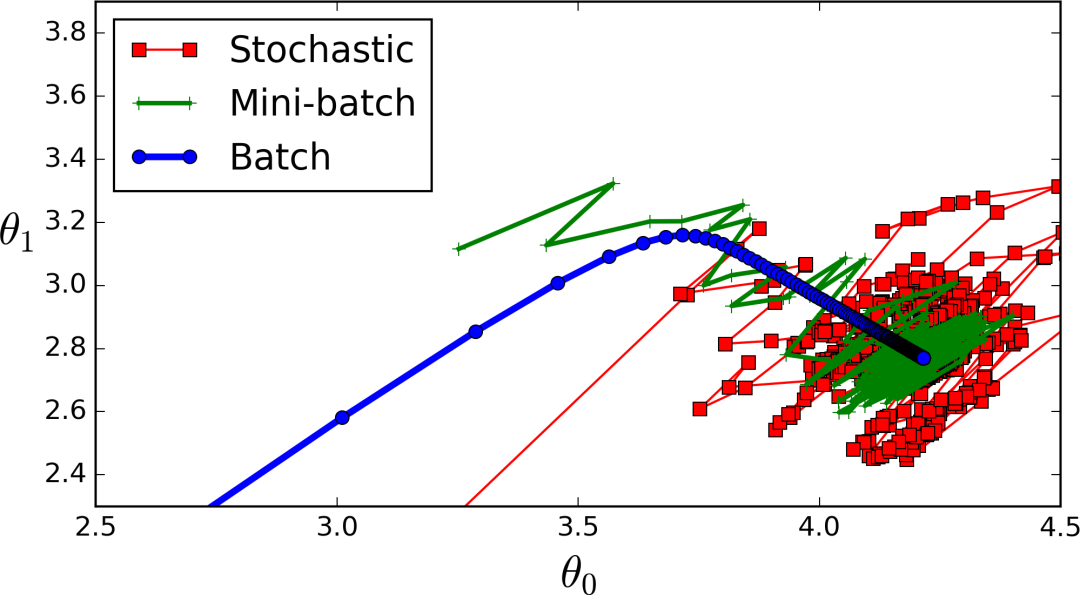梯度下降是数据科学的基础，无论是深度学习还是机器学习。对梯度下降原理的深入了解一定会对你今后的工作有所帮助。

你将真正了解这些超参数的作用、在背后发生的情况以及如何处理使用此算法可能遇到的问题，而不是玩弄超参数并希望获得最佳结果。

然而，梯度下降并不局限于一种算法。另外两种流行的梯度下降（随机和小批量梯度下降）建立在主要算法的基础上，你可能会看到比普通批量梯度下降更多的算法。因此，我们也必须对这些算法有一个坚实的了解，因为它们有一些额外的超参数，当我们的算法没有达到我们期望的性能时，我们需要理解和分析这些超参数。

虽然理论对于深入理解手头的算法至关重要，但梯度下降的实际编码及其不同的“变体”可能是一项困难的任务。为了完成这项任务，本文的格式如下：

1. 简要概述每种算法的作用。

2. 算法的代码

3. 对规范不明确部分的进一步解释

我们将使用著名的波士顿住房数据集，它是预先内置在scikit learn中的。我们还将从头开始构建一个线性模型

好的，首先让我们做一些基本的导入。我不打算在这里做EDA，因为这不是我们文章的真正目的。不过，我会把一些事情说明白。

import numpy as np
import pandas as pd
import plotly.express as px
from sklearn.datasets import load_boston
from sklearn.metrics import mean_squared_error

好的，为了让我们看到数据是什么样子，我将把数据转换成一个数据帧并显示输出。

data = load_boston()

df = pd.DataFrame(data['data'],columns=data['feature_names'])
df.insert(13,'target',data['target'])
df.head(5)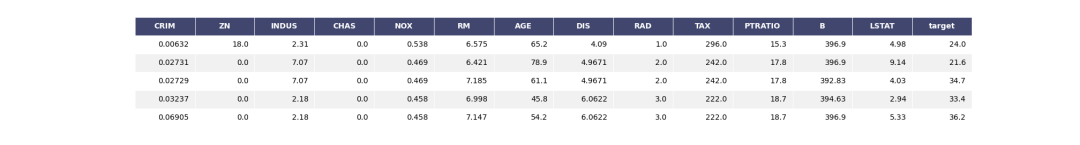好吧，这里没什么特别的，我敢肯定你之前已经类似实现过了。

现在，我们将定义我们的特征（X）和目标（y）。我们还将定义我们的参数向量，将其命名为thetas，并将它们初始化为零。

X,y = df.drop('target',axis=1),df['target']

thetas = np.zeros(X.shape)

### 成本函数

回想一下，成本函数是衡量模型性能的东西，也是梯度下降的目标。我们将使用的代价函数称为均方误差。公式如下：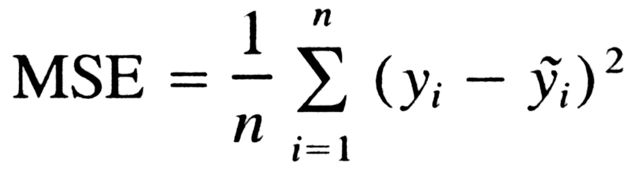好吧，我们把它写出来：

def cost_function(X,Y,B):
predictions = np.dot(X,B.T)

cost = (1/len(Y)) * np.sum((predictions - Y) ** 2)
return cost

在这里，我们将输入、标签和参数作为输入，并使用线性模型进行预测，得到成本，然后返回。如果第二行让你困惑，回想一下线性回归公式：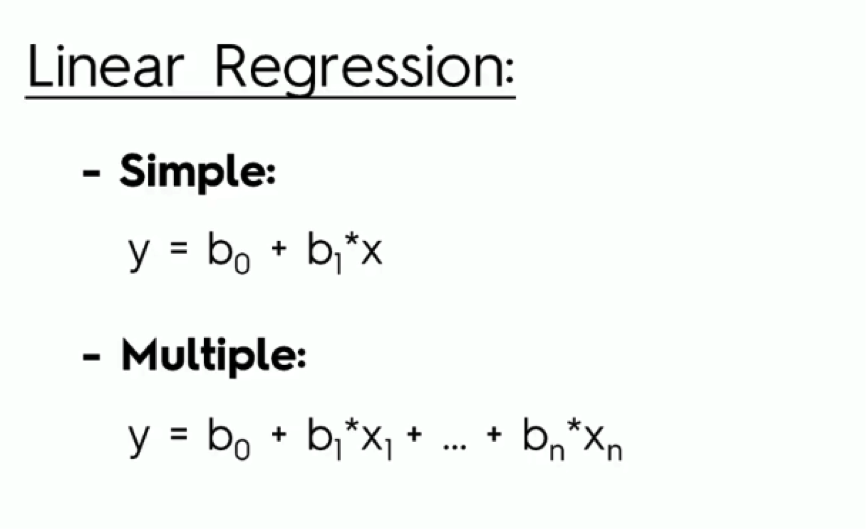很好，现在让我们测试一下我们的成本函数，看看它是否真的有效。为了做到这一点，我们将使用scikit learn的均方误差，得到结果，并将其与我们的算法进行比较。

mean_squared_error(np.dot(X,thetas.T),y)

OUT: 592.14691169960474

cost_function(X,y,thetas)

OUT: 592.14691169960474

太棒了，我们的成本函数起作用了！

### 特征缩放

特征缩放是线性模型（线性回归、KNN、SVM）的重要预处理技术。本质上，特征被缩小到更小的范围，并且特征也在一定的范围内。可以这样考虑特征缩放：

1. 你有一座很大的建筑物

2. 你希望保持建筑的形状，但希望将其调整为较小的比例

特征缩放通常用于以下场景：

1. 如果一个算法使用欧几里德距离，那么由于欧几里德距离对较大的量值敏感，因此需要对特征进行缩放

2. 特征缩放还可以用于数据标准化

3. 特征缩放还可以提高算法的速度

虽然有许多不同的特征缩放方法，但我们将使用以下公式构建MinMaxScaler的自定义实现：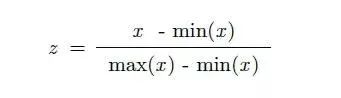由于上述原因，我们将使用缩放。

现在，对于python实现：

X_norm = (X - X.min()) / (X.max() - X.min())
X = X_norm

这里没什么特别的，我们只是把公式翻译成代码。现在，节目真正开始了：梯度下降！### 梯度下降具体地说，梯度下降是一种优化算法，它通过迭代遍历数据并获得偏导数来寻求函数的最小值（在我们的例子中是MSE）。

如果这有点复杂，试着把梯度下降想象成是一个人站在山顶上，他们试着以最快的速度从山上爬下来，沿着山的负方向不断地“走”，直到到达底部。

现在，梯度下降有不同的版本，但是你会遇到最多的是：

1. 批量梯度下降

2. 随机梯度下降法

3. 小批量梯度下降

现在我们将按顺序讨论、实现和分析每一项，所以让我们开始吧！

### 批量梯度下降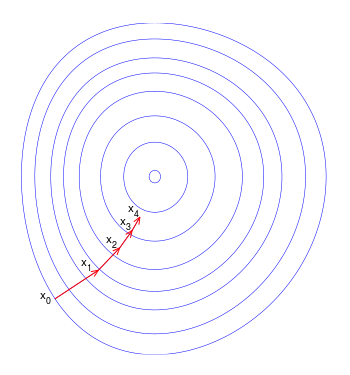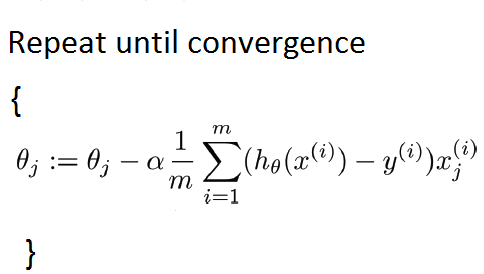我们的数据集很小，所以我们可以像这样实现批量梯度下降：

def batch_gradient_descent(X,Y,theta,alpha,iters):
cost_history =  * iters  # 初始化历史损失列表
for i in range(iters):
prediction = np.dot(X,theta.T)
theta = theta - (alpha/len(Y)) * np.dot(prediction - Y,X)
cost_history[i] = cost_function(X,Y,theta)
return theta,cost_history

要澄清一些术语：

alpha：这是指学习率。

iters：迭代运行的数量。

太好了，现在让我们看看结果吧！

batch_theta,batch_history=batch_gradient_descent(X,y,theta,0.05,500)

好吧，不是很快，但也不是很慢。让我们用我们新的和改进的参数来可视化和成本：

cost_function(X,y,batch_theta)

OUT: 27.537447130784262

哇，从592到27！这只是一个梯度下降的力量的一瞥！让我们对迭代次数的成本函数进行可视化：

fig = px.line(batch_history,x=range(5000),y=batch_history,labels={'x':'no. of iterations','y':'cost function'})
fig.show()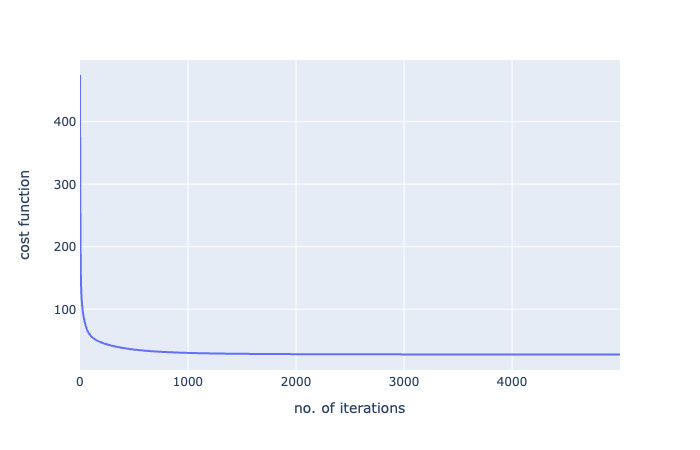好的，看看这个图表，我们在大约100次迭代之后达到了一个大的下降，从那里开始，它一直在逐渐减少。

所以，批量梯度下降到此结束：

#### 优点

1. 有效且曲线平滑

2. 最准确，最有可能达到全局最低值

#### 缺点

1. 对于大型数据集可能会很慢

2. 计算成本高

### 随机梯度下降法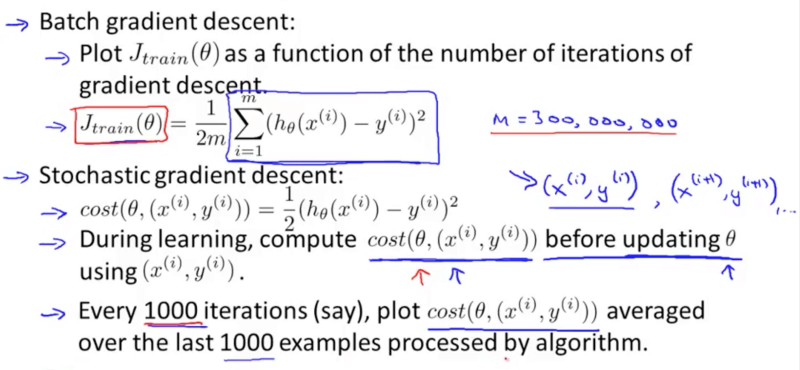这里，不是计算整个训练集的偏导数，而是只计算一个随机样本（随机意义上的随机）。

这是很好的，因为计算只需要在一个训练示例上进行，而不是在整个训练集上进行，这使得计算速度更快，而且对于大型数据集来说非常理想。

然而，由于其随机性，随机梯度下降并不像批量梯度下降那样具有平滑的曲线，虽然它可以返回良好的参数，但不能保证达到全局最小值。

#### 学习率调整

解决随机梯度下降问题的一种方法是学习率调整。

基本上，这会逐渐降低学习率。因此，学习率一开始很大（这有助于避免局部极小值），当学习率接近全局最小值时，学习率逐渐降低。但是，你必须小心：

1. 如果学习速率降低得太快，那么算法可能会陷入局部极小，或者在达到最小值的一半时停滞不前。

2. 如果学习速率降低太慢，可能会在很长一段时间内跳转到最小值附近，仍然无法得到最佳参数

现在，我们将使用简易的学习率调整策略实现随机梯度下降：

t0,t1 = 5,50 # 学习率超参数

def learning_schedule(t):
return t0/(t+t1)

c_hist =  * n_epochs # 历史成本
for epoch in range(n_epochs):
for i in range(len(y)):
random_index = np.random.randint(len(Y))
xi = X[random_index:random_index+1]
yi = y[random_index:random_index+1]

prediction = xi.dot(thetas)

gradient = 2 * xi.T.dot(prediction-yi)
eta = learning_schedule(epoch * len(Y) + i)
thetas = thetas - eta * gradient
c_hist[epoch] = cost_function(xi,yi,thetas)
return thetas,c_hist

现在运行函数：

sdg_thetas,sgd_cost_hist = stochastic_gradient_descent(X,Y,theta)

好吧，太好了，这样就行了！现在让我们看看结果：

cost_function(X,y,sdg_thetas)

OUT:
29.833230764634493

哇！我们从592到29，但是请注意：我们只进行了30次迭代。批量梯度下降，500次迭代后得到27次！这只是对随机梯度下降的非凡力量的一瞥。

让我们用一个图再次将其可视化：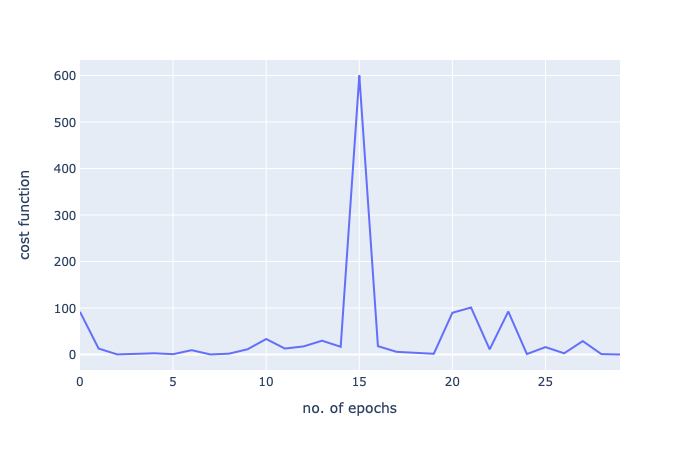由于这是一个小数据集，批量梯度下降就足够了，但这只是显示了随机梯度下降的力量。

#### 优点：

1. 与批量梯度下降相比更快

2. 更好地处理更大的数据集

#### 缺点：

1. 在某个最小值上很难跳出

2. 并不总是有一个清晰的图，可以在一个最小值附近反弹，但永远不会达到最佳的最小值

#### 小批量梯度下降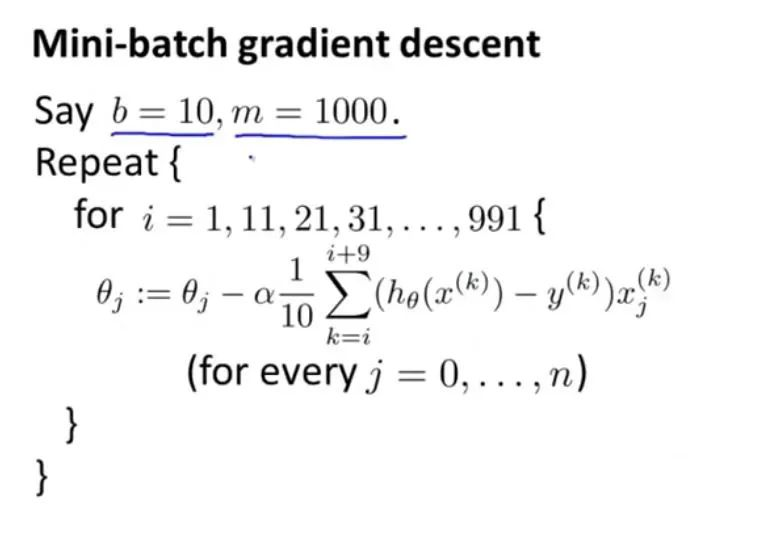好了，快到了，还有一个要通过！现在，在小批量梯度下降中，我们不再计算整个训练集或随机样本的偏导数，而是在整个训练集的小子集上计算。

这给了我们比批量梯度下降更快的速度，因为它不像随机梯度下降那样随机，所以我们更接近于最小值。然而，它很容易陷入局部极小值。

同样，为了解决陷入局部最小值的问题，我们将在实现中使用简易的学习率调整。

np.random.seed(42) # 所以我们得到相同的结果

t0, t1 = 200, 1000
def learning_schedule(t):
return t0 / (t + t1)

t = 0
c_hist =  * n_iters
for epoch in range(n_iters):
shuffled_indices = np.random.permutation(len(y))
X_shuffled = X_scaled[shuffled_indices]
y_shuffled = y[shuffled_indices]

for i in range(0,len(Y),batch_size):
t+=1
xi = X_shuffled[i:i+batch_size]
yi = y_shuffled[i:i+batch_size]

gradient = 2/batch_size * xi.T.dot(xi.dot(thetas) - yi)
eta = learning_schedule(t)
thetas = thetas - eta * gradient
c_hist[epoch] = cost_function(xi,yi,thetas)
return thetas,c_hist

让我们运行并获得结果：

mini_batch_gd_thetas,mini_batch_gd_cost = mini_batch_gradient_descent(X,y,theta)

以及新参数下的成本函数：

cost_function(X,Y,mini_batch_gd_thetas)

OUT: 27.509689139167012

又一次真的很棒。我们运行了1/5的迭代，我们得到了一个更好的分数！

让我们再画出函数：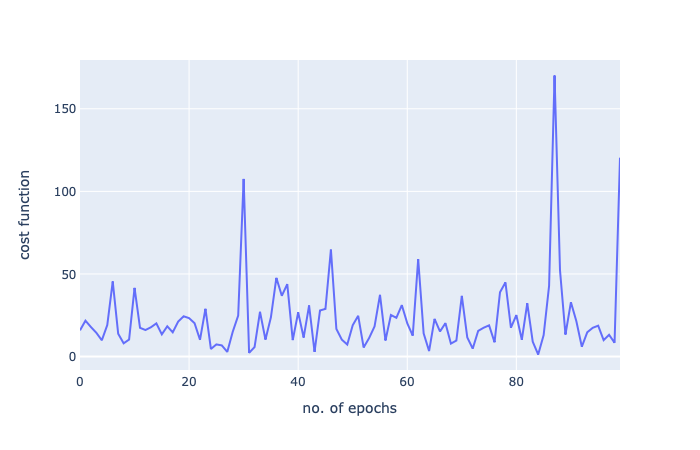好了，我的梯度下降系列到此结束！感谢阅读！

好消息！

小白学视觉知识星球

开始面向外开放啦👇👇👇下载1：OpenCV-Contrib扩展模块中文版教程

在「小白学视觉」公众号后台回复：扩展模块中文教程，即可下载全网第一份OpenCV扩展模块教程中文版，涵盖扩展模块安装、SFM算法、立体视觉、目标跟踪、生物视觉、超分辨率处理等二十多章内容。

下载2：Python视觉实战项目52讲
在「小白学视觉」公众号后台回复：Python视觉实战项目，即可下载包括图像分割、口罩检测、车道线检测、车辆计数、添加眼线、车牌识别、字符识别、情绪检测、文本内容提取、面部识别等31个视觉实战项目，助力快速学校计算机视觉。

下载3：OpenCV实战项目20讲
在「小白学视觉」公众号后台回复：OpenCV实战项目20讲，即可下载含有20个基于OpenCV实现20个实战项目，实现OpenCV学习进阶。

交流群

欢迎加入公众号读者群一起和同行交流，目前有SLAM、三维视觉、传感器、自动驾驶、计算摄影、检测、分割、识别、医学影像、GAN、算法竞赛等微信群（以后会逐渐细分），请扫描下面微信号加群，备注：”昵称+学校/公司+研究方向“，例如：”张三 + 上海交大 + 视觉SLAM“。请按照格式备注，否则不予通过。添加成功后会根据研究方向邀请进入相关微信群。请勿在群内发送广告，否则会请出群，谢谢理解~
展开全文• 机器学习中经典的优化算法，让loss快速达到更小，本代码在minist数据集测试分类算法，取得很好的结果。
• python版本选择 这里选的python版本是2.7，因为我之前用python3试了几次，发现在画3d图的时候会报错，所以改用了2.7。 数据集选择 数据集我选了一个包含两个变量，三个参数的数据集，这样可以画出3d图形对结果进行...
• 目录 全量梯度下降 随机梯度下降 ...全量梯度下降每次学习都使用整个训练集，因此其优点在 于每次更新都会朝着正确的方向进行，最后能够保证收敛于极值点(凸函数收敛于全局极值 点，非凸函数可能会收敛于局

目录

全量梯度下降

随机梯度下降

小批量梯度下降

三种梯度下降区别和优缺点

# 全量梯度下降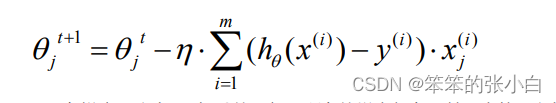在梯度下降中，对于θ的更新，所有的样本都有贡献，也就是参与调整θ。其计算得到 的是一个标准梯度。因而理论上来说一次更新的幅度是比较大的。如果样本不多的情况下， 当然是这样收敛的速度会更快啦。全量梯度下降每次学习都使用整个训练集，因此其优点在 于每次更新都会朝着正确的方向进行，最后能够保证收敛于极值点(凸函数收敛于全局极值 点，非凸函数可能会收敛于局部极值点)，但是其缺点在于每次学习时间过长，并且如果训 练集很大以至于需要消耗大量的内存，并且全量梯度下降不能进行在线模型参数更新。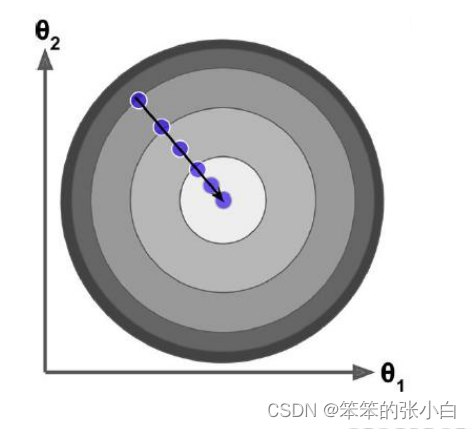代码实现：

import numpy as np

# 创建数据集X，y
np.random.seed(1)
X = np.random.rand(100, 1)
y = 4 + 3*X + np.random.randn(100, 1)
X_b = np.c_[np.ones((100, 1)), X]

# 创建超参数
n_iterations = 10000
t0, t1 = 5, 500

# 定义一个函数来动态调整学习率
def learning_rate_schedule(t):
return t0/(t+t1)

# 1,初始化θ, W0...Wn，标准正太分布创建W
theta = np.random.randn(2, 1)
# 4,判断是否收敛，一般不会去设定阈值，而是直接采用设置相对大的迭代次数保证可以收敛
for i in range(n_iterations):
learning_rate = learning_rate_schedule(i)
theta = theta - learning_rate * gradients
print(theta)

[[4.23695725]
[2.68492509]]

# 随机梯度下降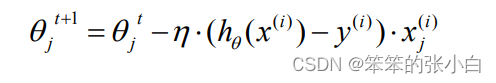梯度下降算法每次从训练集中随机选择一个样本来进行学习。批量梯度下降算法每次都 会使用全部训练样本，因此这些计算是冗余的，因为每次都使用完全相同的样本集。而随机 梯度下降算法每次只随机选择一个样本来更新模型参数，因此每次的学习是非常快速的，并 且可以进行在线更新。随机梯度下降最大的缺点在于每次更新可能并不会按照正确的方向进 行，因此可以带来优化波动(扰动)。

不过从另一个方面来看，随机梯度下降所带来的波动有个好处就是，对于类似盆地区域 （即很多局部极小值点）那么这个波动的特点可能会使得优化的方向从当前的局部极小值点 跳到另一个更好的局部极小值点，这样便可能对于非凸函数，最终收敛于一个较好的局部极 值点，甚至全局极值点。由于波动，因此会使得迭代次数（学习次数）增多，即收敛速度变 慢。不过最终其会和全量梯度下降算法一样，具有相同的收敛性，即凸函数收敛于全局极值 点，非凸损失函数收敛于局部极值点。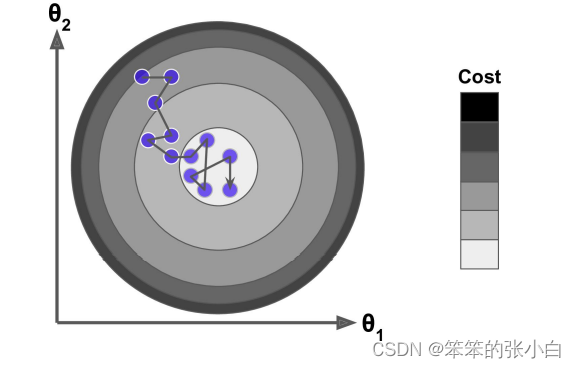代码实现：

import numpy as np

# 创建数据集
X = 2*np.random.rand(100, 1)
y = 4 + 3*X + np.random.randn(100, 1)
X_b = np.c_[np.ones((100, 1)), X]
# 创建超参数
n_epochs = 10000
m = 100
t0, t1 = 5, 500

# 定义一个函数来调整学习率
def learning_rate_schedule(t):
return t0/(t+t1)

theta = np.random.randn(2, 1)
for epoch in range(n_epochs):
# 在双层for循环之间，每个轮次开始分批次迭代之前打乱数据索引顺序
arr = np.arange(len(X_b))
np.random.shuffle(arr)
X_b = X_b[arr]
y = y[arr]
for i in range(m):
xi = X_b[i:i+1]
yi = y[i:i+1]
learning_rate = learning_rate_schedule(epoch*m + i)
theta = theta - learning_rate * gradients

print(theta)

[[3.91306085]
[3.16087742]]

# 小批量梯度下降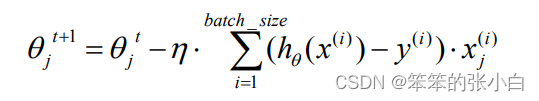Mini-batch 梯度下降综合了 batch 梯度下降与 stochastic 梯度下降，在每次更新速 度与更新次数中间取得一个平衡，其每次更新从训练集中随机选择 batch_size，batch_size < m 个样本进行学习。相对于随机梯度下降算法，小批量梯度下降算法降低了收敛波动性， 即降低了参数更新的方差，使得更新更加稳定。相对于全量梯度下降，其提高了每次学习的速度。并且其不用担心内存瓶颈从而可以利用矩阵运算进行高效计算。一般而言每次更新随 机选择[50,256]个样本进行学习，但是也要根据具体问题而选择，实践中可以进行多次试验， 选择一个更新速度与更次次数都较适合的样本数。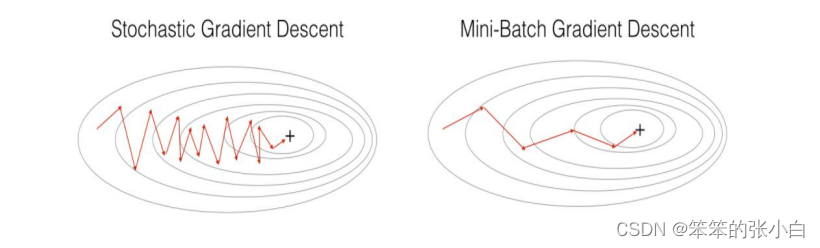代码实现：

import numpy as np

# 创建数据集X，y
X = 2*np.random.rand(100, 1)
y = 4 + 3*X + np.random.randn(100, 1)
X_b = np.c_[np.ones((100, 1)), X]

# 创建超参数
t0, t1 = 5, 500

# 定义一个函数来动态调整学习率
def learning_rate_schedule(t):
return t0/(t+t1)

n_epochs = 100000
m = 100
batch_size = 10
num_batches = int(m / batch_size)

theta = np.random.randn(2, 1)
for epoch in range(n_epochs):
arr = np.arange(len(X_b))
np.random.shuffle(arr)
X_b = X_b[arr]
y = y[arr]
for i in range(num_batches):
x_batch = X_b[i*batch_size: i*batch_size + batch_size]
y_batch = y[i*batch_size: i*batch_size + batch_size]
learning_rate = learning_rate_schedule(epoch * m + i)
theta = theta - learning_rate*gradients

print(theta)

[[3.91630905]
[2.95252566]]

# 三种梯度下降区别和优缺点

在讲三种梯度下降区别之前，我们先来总结一下梯度下降法的步骤：

1. 瞎蒙，Random 随机θ，随机一组数值 W0…Wn

2. 求梯度，为什么是梯度？因为梯度代表曲线某点上的切线的斜率，沿着切线往下下降就 相当于沿着坡度最陡峭的方向下降

3. if g0, theta 往小调

4. 判断是否收敛 convergence，如果收敛跳出迭代，如果没有达到收敛，回第 2 步继续 四步骤对应计算方式：

a. np.random.rand()或者 np.random.randn()

b. i w j gradient = (h (x) - y)× x

c. Wi t+1 =Wi t -h ×gradient i

d. 判断收敛这里使用 g=0 其实并不合理，因为当损失函数是非凸函数的话 g=0 有可能是 极大值对吗！所以其实我们判断 loss 的下降收益更合理，当随着迭代 loss 减小的幅度 即收益不再变化就可以认为停止在最低点，收敛！

区别：其实三种梯度下降的区别仅在于第 2 步求梯度所用到的 X 数据集的样本数量不同！ 它们每次学习(更新模型参数)使用的样本个数，每次更新使用不同的样本会导致每次学习的 准确性和学习时间不同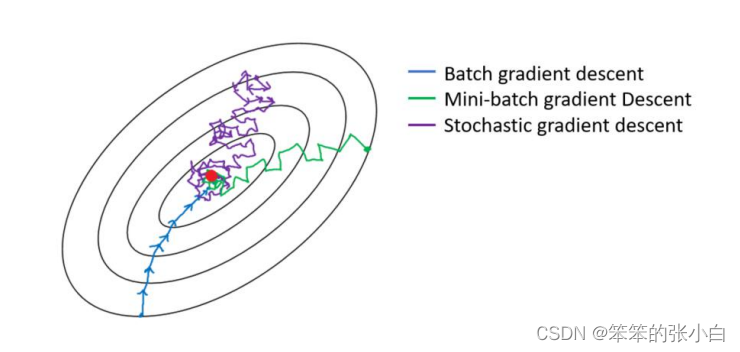展开全文机器学习
• 本文简要介绍了黄金分割法，Armijo线搜索算法，Wolfe-Powell线搜索算法梯度下降法python
• 梯度下降法不是机器学习算法，不能用来解决分类或回归问题，而是一种基于搜索的最优化方法，作用是优化目标函数，如求损失函数的最小值，即梯度下降法。 梯度 梯度的本意是一个向量（矢量），表示某一函数在该点处的...机器学习
• 个人公众号：经管人学数据分析基本介绍梯度下降法(gradient descent)，又名最速下降法(steepest descent)是求解无约束最优化问题最常用的方法，它是一种迭代方法，每一步主要的操作是求解目标函数的梯度向量，将当前...
• 文章目录一、什么是梯度下降法二、如何理解梯度下降法2.1 概念2.2 举例说明 一、什么是梯度下降法 梯度下降是迭代法的一种,可以用于求解最小二乘问题(线性和非线性都可以)。在求解机器学习算法的模型参数，即无约束...
• python机器学习 一元线性回归 梯度下降法实现Python机器学习系列（四）】机器学习 python 线性回归 梯度下降 数据挖掘
• 本文实例为大家分享了python实现梯度下降算法的具体代码，供大家参考，具体内容如下 简介 本文使用python实现梯度下降算法，支持y = Wx+b的线性回归 目前支持批量梯度算法和随机梯度下降算法(bs=1) 也支持输入...
• 主要为大家详细介绍了python实现梯度下降和逻辑回归，文中示例代码介绍的非常详细，具有一定的参考价值，感兴趣的小伙伴们可以参考一下
• 主要为大家详细介绍了python使用梯度下降算法实现一个多线性回归，文中示例代码介绍的非常详细，具有一定的参考价值，感兴趣的小伙伴们可以参考一下
• 批量梯度下降法python具体实现, np.random.seed(666) x = 2 * np.random.random(size = 100) y = x * 3.0 + 4.0 + np.random.normal(size = 100) X = x.reshape(-1, 1) #损失函数 def J(theta, X_b, y): try: ...
• def fun(x, y, w, b): l = 0 for i in range(4): l += (y[i] - (b + w * x[i]))**2 # l = sum(y - (b + w * x)**2 for x, y in zip(x, y)) / 8 return l / 8 # 梯度下降法 def gradient_descent(): times = 100 # ...python 机器学习
• 最终，梯度下降算法： 学习率一般小于1，当损失函数是0时，我们输出theta0和theta1. 接下来上代码！ class LinearRegression(): def __init__(self, data, theta0, theta1, learning_rate): self.data = ...
• 在上一篇逻辑回归中，我们利用批量梯度下降算法BGD求解使损失函数J(θ)取得最小值的θ，同时也提到了SGD和MBGD，...1. 批量梯度下降法(Batch Gradient Descent)批量梯度下降算法是梯度下降算法的最常用形式，即在更...
• ①概述 不是一个机器学习算法 是一种基于搜索的最优化方法 作用：最小化一个损失函数 梯度上升法：最大化一个效用函数 思路：由上图可知，在损失函数上的某一个点，希望经过变化到达损失函数... 是梯度下降法python pycharm 机器学习 sklearn
• 梯度下降和随机梯度下降的区别是，梯度下降在每次更新时，使用所有样本来计算，这样的实现...python实现随机梯度下降如下，使用常规的线性模型来测试效果。随机梯度下降指的是用样本中的一个例子来代替所有的样本，...python 机器学习
• 梯度下降法代码python实现 -有数据有图有真相 今天我们做了一个实验，通过生成多元回归数据进行梯度下降求解权重 生成一份线性数据 y=w1∗x1=w2∗x2+w3∗x3+by=w_1*x_1=w_2*x_2+w_3*x_3+by=w1​∗x1​=w2​∗x2​+...python 机器学习
• 在学习线性回归的时候很多课程都会讲到用梯度下降法求解参数，对于梯度下降算法怎么求出这个解讲的较少，自己实现一遍算法比较有助于理解算法，也能注意到比较细节的东西。具体的数学推导可以参照这一篇博客...梯度下降 随机梯度下降
• 刚开始学习机器学习，了解了代价函数，梯度下降算法，用一组最简单的数据进行拟合 对于该算法的自己的一些理解 我们在拟合的过程中，需要一个判断拟合准确程度的函数，这个函数就叫损失函数（也叫做代价函数） 例：...python 机器学习...